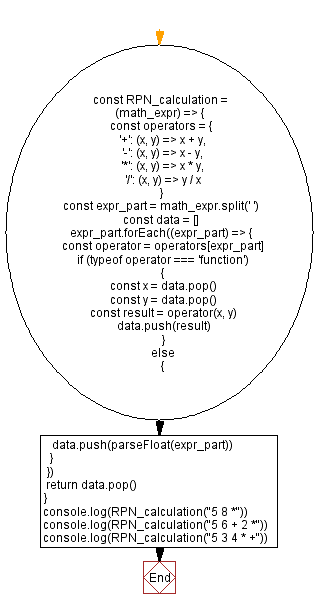# JavaScript: Reverse Polish notation in mathematical expression

## JavaScript Math: Exercise-70 with Solution

Write a JavaScript program to apply reverse Polish notation to a given mathematical expression.

From Wikipedia -
Reverse Polish notation (RPN), also known as reverse Łukasiewicz notation, Polish postfix notation or simply postfix notation, is a mathematical notation in which operators follow their operands, in contrast to Polish notation (PN), in which operators precede their operands. It does not need any parentheses as long as each operator has a fixed number of operands. The description "Polish" refers to the nationality of logician Jan Łukasiewicz, who invented Polish notation in 1924.

Sample Data:
"5 8 *" -> 40
"5 6 + 2 *" -> 22
"5 3 4 * +" -> 17

Sample Solution:

HTML Code:

``````<!DOCTYPE html>
<html>
<meta charset="utf-8">
<title>JavaScript function to reverse Polish notation in mathematical expression</title>
<body>

</body>
</html>
```
```

JavaScript Code:

``````const RPN_calculation = (math_expr) => {
const operators = {
'+': (x, y) => x + y,
'-': (x, y) => x - y,
'*': (x, y) => x * y,
'/': (x, y) => y / x
}
const expr_part = math_expr.split(' ')
const data = []

expr_part.forEach((expr_part) => {
const operator = operators[expr_part]
if (typeof operator === 'function')
{
const x = data.pop()
const y = data.pop()
const result = operator(x, y)
data.push(result)
}
else
{
data.push(parseFloat(expr_part))
}
})
return data.pop()
}
console.log(RPN_calculation("5 8 *"))
console.log(RPN_calculation("5 6 + 2 *"))
console.log(RPN_calculation("5 3 4 * +"))
```
```

Sample Output:

```40
22
17
```

Flowchart:Live Demo:

See the Pen javascript-math-exercise-70 by w3resource (@w3resource) on CodePen.

Improve this sample solution and post your code through Disqus

What is the difficulty level of this exercise?

Test your Programming skills with w3resource's quiz.

﻿

## JavaScript: Tips of the Day

```const user = { name: 'Owen', age: 21 };